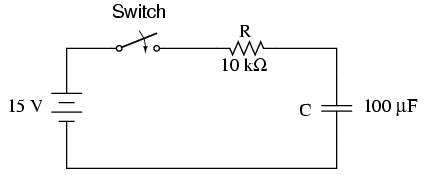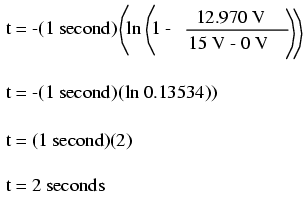16.8: Solving for Unknown Time

Sometimes it is necessary to determine the length of time that a reactive circuit will take to reach a predetermined value. This is especially true in cases where we’re designing an RC or L/R circuit to perform a precise timing function. To calculate this, we need to modify our “Universal time constant formula.” The original formula looks like this:However, we want to solve for time, not the amount of change. To do this, we algebraically manipulate the formula so that time is all by itself on one side of the equal sign, with all the rest on the other side:The ln designation just to the right of the time constant term is the natural logarithm function: the exact reverse of taking the power of e. In fact, the two functions (powers of e and natural logarithms) can be related as such:

If ex = a, then ln a = x.

If ex = a, then the natural logarithm of a will give you x: the power that e must be was raised to in order to produce a.

Let’s see how this all works on a real example circuit. Taking the same resistor-capacitor circuit from the beginning of the chapter, we can work “backwards” from previously determined values of voltage to find how long it took to get there.The time constant is still the same amount: 1 second (10 kΩ times 100 µF), and the starting/final values remain unchanged as well (EC = 0 volts starting and 15 volts final). According to our chart at the beginning of the chapter, the capacitor would be charged to 12.970 volts at the end of 2 seconds. Let’s plug 12.970 volts in as the “Change” for our new formula and see if we arrive at an answer of 2 seconds:Indeed, we end up with a value of 2 seconds for the time it takes to go from 0 to 12.970 volts across the capacitor. This variation of the universal time constant formula will work for all capacitive and inductive circuits, both “charging” and “discharging,” provided the proper values of time constant, Start, Final, and Change are properly determined beforehand. Remember, the most important step in solving these problems is the initial set-up. After that, its just a lot of button-pushing on your calculator!

REVIEW:

• To determine the time it takes for an RC or L/R circuit to reach a certain value of voltage or current, you’ll have to modify the universal time constant formula to solve for time instead of change.• The mathematical function for reversing an exponent of “e” is the natural logarithm (ln), provided on any scientific calculator.